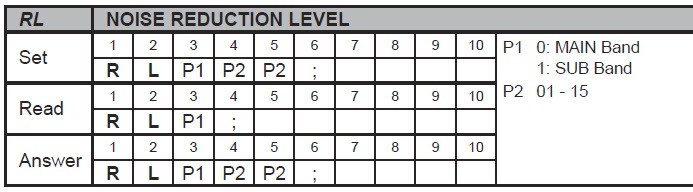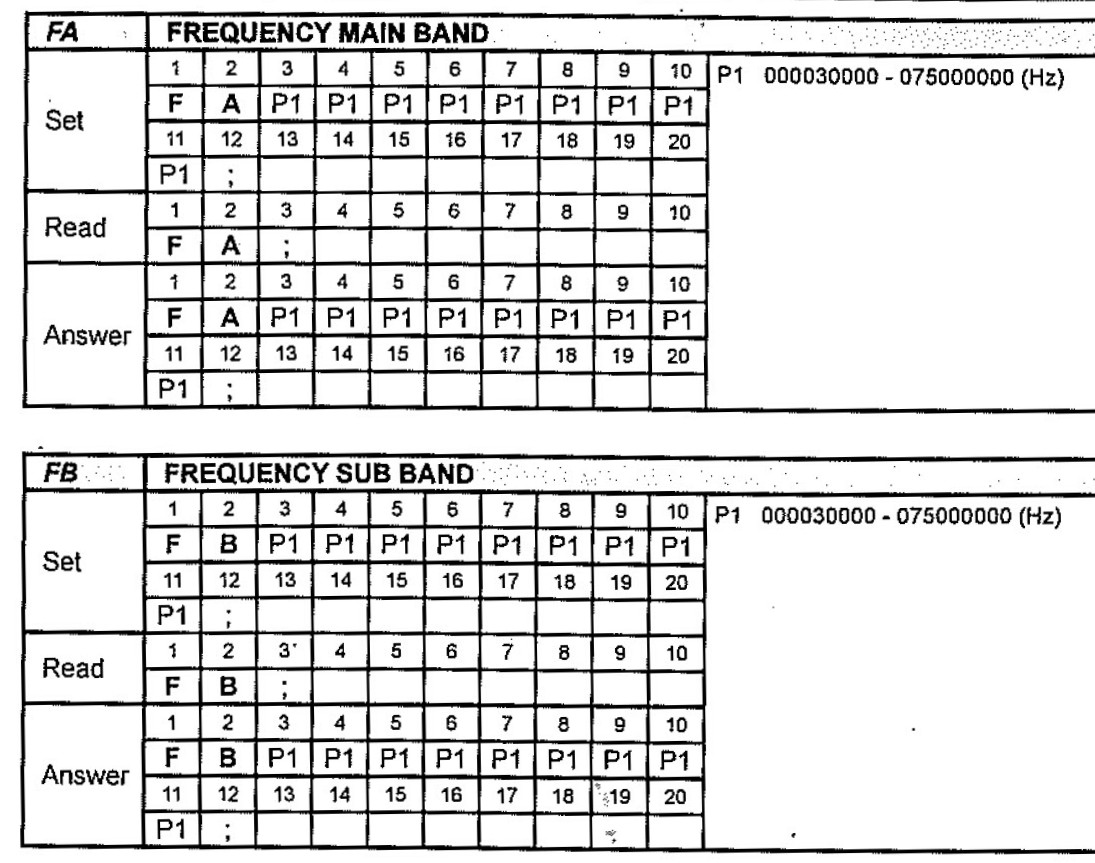The examples are from the FTdx101D. Other modern Yaesu radios have a similar CAT system.

My configuration system for older Yaesu (5 - byte command) radios also uses these command masks.

piWebCAT's control actions are NOT read-modify-write (unlike my EncoderCAT project)
The controls are synchronised to the radio's settings at startup, on band change and by the reload button.

Subsequent button or slider action commands use setmask with the new position of a slider,
or from known buttons states.      ie: only the set command is used.

(eg: for toggled buttons, piWebCAT uses the button's remembered on or off state to determine the new state.)

A example from the FTdx101D CAT manual -  Noise Reduction Level.

The CAT manual entry is below. There are two identical receivers, MAIN and SUB.

(Note that the terminating semicolon is part of the command)• MAIN  -  read = "RL0;"    answer  = "RL006;"   set = "RL007;"
• SUB   -  read = "RL1;"    answer  = "RL106;"   set = "RL107;"

In   MAIN.Answer  above:     "RL0" is the command and '"06' is the variable data.

We configure the masks as follows::

• "RL0" and RL1 are fixed as the first three characters.
• '"tu"  indicates that the fourth and fifth characters are variable tens and units.

readbytes is 4 (ie: RL0; is 4 bytes)    setbytes and answerbytes are 6 (ie: RL0tu; is six bytes)

Further example:   frequency read and write

In piWebCAT, we tune with the current VFO which may be A or B

Yaesu CAT manual statesnumbers 0 - 999 999 999    ie: 0 - 999 Mhz

readbytes is 3 (ie: FA; is 3bytes)    setbytes and answerbytes are both 12.

List of 'variable' characters

I have defined a small list of lower case characters that represent variable data.
All other characters outside this list are interpreted as fixed.

The characters are g, f, e, d, c, m, h, t, u   (u = units,   t= tens, h = hundreds  etc)

There is also s  = sign - representing  '-'  or  '+'

\$ is don't care

The codes are detailed below in an extract from EncoderCAT C source code:

EncoderCAT and piWebCAT use the same codes for character interpretation:

For decimal digit ascii characters, ie:  '0'  to  '9'

switch(mb)

{

case 'g': mult = 100000000; break;        // g = hundred millions

case 'f': mult = 10000000; break;        // f = ten millions

case 'e': mult = 1000000; break;        // e = millions

case 'd': mult = 100000; break;                // d = hundred thousands

case 'c': mult = 10000; break;                // c = ten thousands

case 'm': mult = 1000; break;                // m = thousands

case 'h': mult = 100; break;                // h = hundreds

case 't': mult = 10; break;                // t = tens

case 'u': mult = 1; break;                 // u = units

}

// Also    s represents sign '-' or '+'

There is also  x  which is used for hexdecimal characters.  eg: xx or xxxx